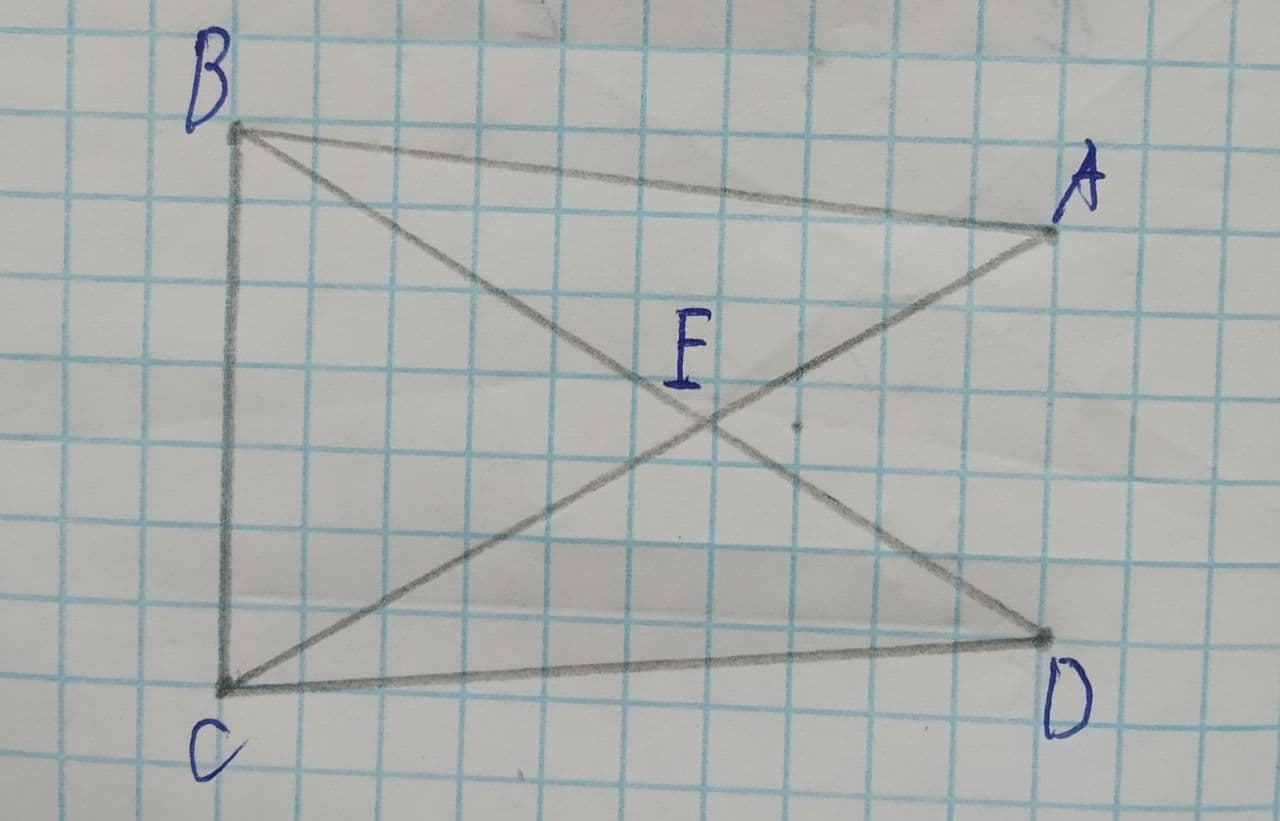Question# Find the congruence criteria \overline{AB}\cong\overline{DC}\ and\ \overline{AC}\cong\overline{DB} used to prove the triangles are congruent

Congruence
ANSWEREDTo find: congruent criteria used to prove that the triangles are congruent.
Given information: $$\displaystyle\overline{{{A}{B}}}\stackrel{\sim}{=}\overline{{{D}{C}}}\ {\quad\text{and}\quad}\ \overline{{{A}{C}}}\stackrel{\sim}{=}\overline{{{D}{B}}}$$2021-07-14
Consider $$\displaystyle\triangle{A}{B}{C},\triangle{D}{C}{B}$$
$$\displaystyle\overline{{{A}{B}}}\stackrel{\sim}{=}\overline{{{D}{C}}}$$ (Given)
$$\displaystyle\overline{{{A}{C}}}\stackrel{\sim}{=}\overline{{{D}{B}}}$$ (Given)
$$\displaystyle\overline{{{B}{C}}}\stackrel{\sim}{=}\overline{{{C}{B}}}$$ (common side of $$\displaystyle\triangle{A}{B}{C},\triangle{D}{C}{B}$$)
Therefore,
$$\displaystyle\triangle{A}{B}{C}\stackrel{\sim}{=}\triangle{D}{C}{B}$$ (SSS congruence criteria)
Also.
Congruent triangles basically overlap each other.
So, $$\displaystyle\triangle{A}{B}{C},\triangle{D}{C}{B}$$ is a pair of overlapping triangles.